# Chapter 3 The Quest for Causality

## 3.1 Introduction

In order to familiarize you with the R code necessary to complete the assignments in Real Econometrics, I will reproduce all examples from Chapter 1. As I present the examples, I will explain the syntax for each pieced of code. You will also be introduced to using R Markdown to produce a seamless integration of your code, your output, and your reports.

In subsequent chapters, I will take you through examples of the relevant code necessary to complete the exercises in R.

### 3.1.1 Table 1.1

Table 1.1 contains the necessary information to produce Figures 1.2 and 1.3. Creating Table 1.1 will give you an opportunity to create a data frame from four vectors. A data frame is used for storing data tables. A data frame is one of the many data structures in R. The others include vector, list, matrix, factors, and tables. A data frame is collection of vectors of the same length.

A vector is the most common and basic data structure in R. Vectors can be of two types: atomic vectors or lists. An atomic vector is a collection of observations of a single variable. The vectors in a data frame can be different types, however. Each vector must be of a single type. The atomic vector types or classes in R are logical, integer, numeric (real or decimal), complex, and character. A logical vector is one in which all of the values are TRUE, FALSE, and NA. An integer vector contains only integers, a real vector contains only reals, etc. If a vector contains more than one type of value, the vector and each element of it is coerced to the most general class in the vector.

Let’s start by creating each vector in Table 1.1. To assign values to a vector, use the assignment operator <- and the concatenate or combine function c().

observation_number <- c(1:13) # The colon tells R to create a sequence from 1 to 13 by 1.  This a vector of integers.
name <- c("Homer", "Marge", "Lisa", "Bart", "Comic Book Guy", "Mr. Burns",
"Smithers", "Chief Wiggum", "Principle Skinner", "Rev. Lovejoy",
"Ned Flanders", "Patty", "Selma")  # Each "string" is enclosed in quotes.  This is a character vector.
donuts_per_week <- c(14, 0, 0, 5, 20, 0.75, 0.25, 16, 3, 2, 0.8, 5, 4) # This is a numeric vector.
weight <- c(275, 141, 70, 75, 310, 80, 160, 263, 205, 185, 170, 155, 145) # This is a numeric vector.

Note in the code chunk above that the symbol, #, is used to create comments within the code. Those things set off by the # will not be executed as code. These are useful for creating notes to yourself or collaborators about what you are doing with certain lines of code.

We now have four named vectors that we can put into a data frame. A note on naming conventions in R. While there are many name conventions in R, I recommend using snake case where each word is separated by an under score and no capital letters are used. See Hadley Wickhams Style Guide for style suggestions for all parts of R programming, not just variable names. Following these guidelines will make your code easier to read and edit.

library(tidyverse) # load the tidyverse package
donuts <- tibble(observation_number, name, donuts_per_week, weight) # create the donuts tibble
save(donuts, file = "donuts.RData")

A tibble is an update to the traditional data frame. For most of what we will do, it will act the same as a data frame. The two main differences in data frames and tibbles are printing and subsetting. For more on tibbles type vignette("tibble") in the console.

tidyverse is one of the many packages developed within the R community. In R, a package is shareable code that bundles together code, data, documentation, tests, etc. To use a package, it must first be installed and then be loaded. To install a package, call install.packages("package_name")2. To make use of a package, load it by calling library(packagename)3. Currently there are more than 14,000 packages available, to see the packages visit Contributed Packages. CRAN Task Views shows relevant packages by task. You may want to visit CRAN Task View: Econometrics to see the extensive array of packages for use in econometrics.

The tidyverse package is a collection of packages that share an underlying design philosophy, grammar, and data structures. For more on the tidyverse follow this link. The dplyr package loaded below is a grammar of data manipulation that can be used to solve most data manipulation problems.

# Print the tibble to the console by typing its name.
donuts
# A tibble: 13 x 4
observation_number name              donuts_per_week weight
<int> <chr>                       <dbl>  <dbl>
1                  1 Homer                       14       275
2                  2 Marge                        0       141
3                  3 Lisa                         0        70
4                  4 Bart                         5        75
5                  5 Comic Book Guy              20       310
6                  6 Mr. Burns                    0.75     80
7                  7 Smithers                     0.25    160
8                  8 Chief Wiggum                16       263
9                  9 Principle Skinner            3       205
10                 10 Rev. Lovejoy                 2       185
11                 11 Ned Flanders                 0.8     170
12                 12 Patty                        5       155
13                 13 Selma                        4       145
# glimpse will provide information about the data frame, its observations, variables, and their class.
library(dplyr)
glimpse(donuts)
Observations: 13
Variables: 4
$observation_number <int> 1, 2, 3, 4, 5, 6, 7, 8, 9, 10, 11, 12, 13$ name               <chr> "Homer", "Marge", "Lisa", "Bart", "Comic Bo...
$donuts_per_week <dbl> 14.00, 0.00, 0.00, 5.00, 20.00, 0.75, 0.25,...$ weight             <dbl> 275, 141, 70, 75, 310, 80, 160, 263, 205, 1...

Use the kable function in knitr to create Table 1.1.

knitr::kable(donuts,
caption = 'Table 1.1 Donut Consumption and Weight',
col.names = c("Observation</br> number", # </br> is html code to insert a line break
"Name", "Donuts</br> per week",
"Weight</br> (pounds)"),
escape = F,  # necessary to force the line breaks
align = 'cccc') # request that the four columns be centered
Table 3.1: Table 1.1 Donut Consumption and Weight
Observation
number
Name Donuts
per week
Weight
(pounds)
1 Homer 14.00 275
2 Marge 0.00 141
3 Lisa 0.00 70
4 Bart 5.00 75
5 Comic Book Guy 20.00 310
6 Mr. Burns 0.75 80
7 Smithers 0.25 160
8 Chief Wiggum 16.00 263
9 Principle Skinner 3.00 205
10 Rev. Lovejoy 2.00 185
11 Ned Flanders 0.80 170
12 Patty 5.00 155
13 Selma 4.00 145

### 3.1.2 Figure 1.2

To create Figure 1.2 we will use the ggplot2 package. ggplot2, also part of the tidyverse, is a system for declarative creating graphics, based on The Grammar of Graphics. The Grammar of Graphics is built on two principles. First, graphics are built with distinct layers of grammatical elements. Second, meaningful plots are formed through aesthetic mappings.

Seven elements comprise the grammar of graphics: data, aesthetics, geometries, facets, statistics, coordinates, and themes. Every graphic must contain, at a minimum, data, aesthetics, and geometries. Data, typically a data frame or tibble, is the data set being plotted. Aesthetics are the scales onto which data are mapped. Aesthetics include x-axis, y-axis, color, fill, size, labels, alpha (transparency), shape, line width, and line type. Geometries are how we want the data plotted, e.g., as points, lines, bars, histograms, boxplots, etc. Facets allow us to use more than one plot, statistics allow us to add elements like error bands, regression lines, etc. Coordinates allow us to control the space into which we plot the data. Finally, themes are all non-data ink in a graphic.

# Load the ggplot2 library
library(ggplot2)
# Create an object p which includes the data and aesthetic mapping
p <- ggplot(data = donuts, mapping = aes(x = donuts_per_week, y = weight))
# Add the geometry that creates the scatter plot
(p1 <- p + geom_point()) # putting parenthesis around the line of code force the output to the screen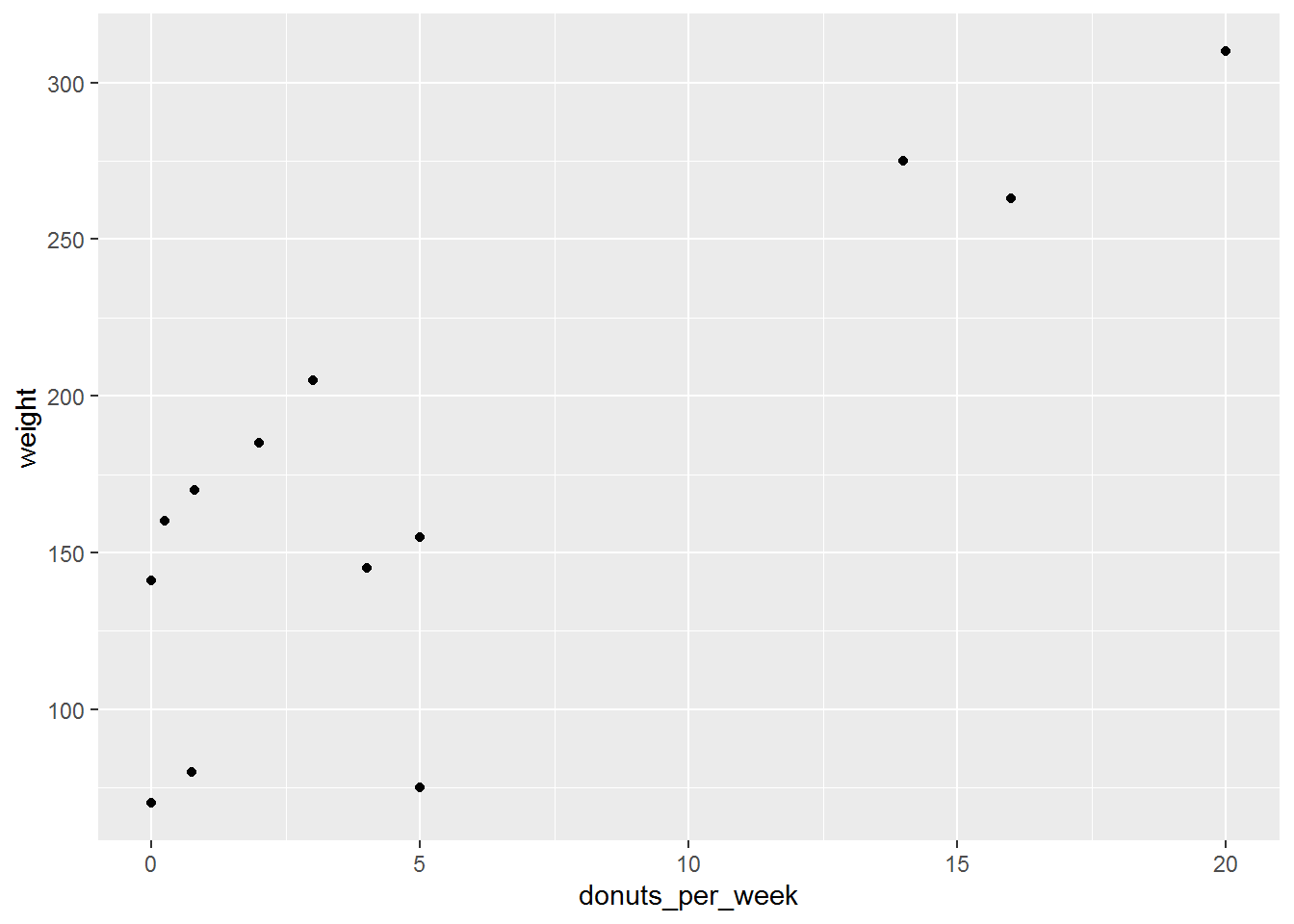# the parentheses surrounding the function call cause the output to be printed.

This basic plot can be transformed into the figure in the text by adding layers to the graphic to change its appearance.

# Change the axis labels and add a caption
(p2 <- p1 + labs(x = "Donuts", y = "Weight (in pounds)", caption = "Figure 1.2: Weight and Donuts in Springfield"))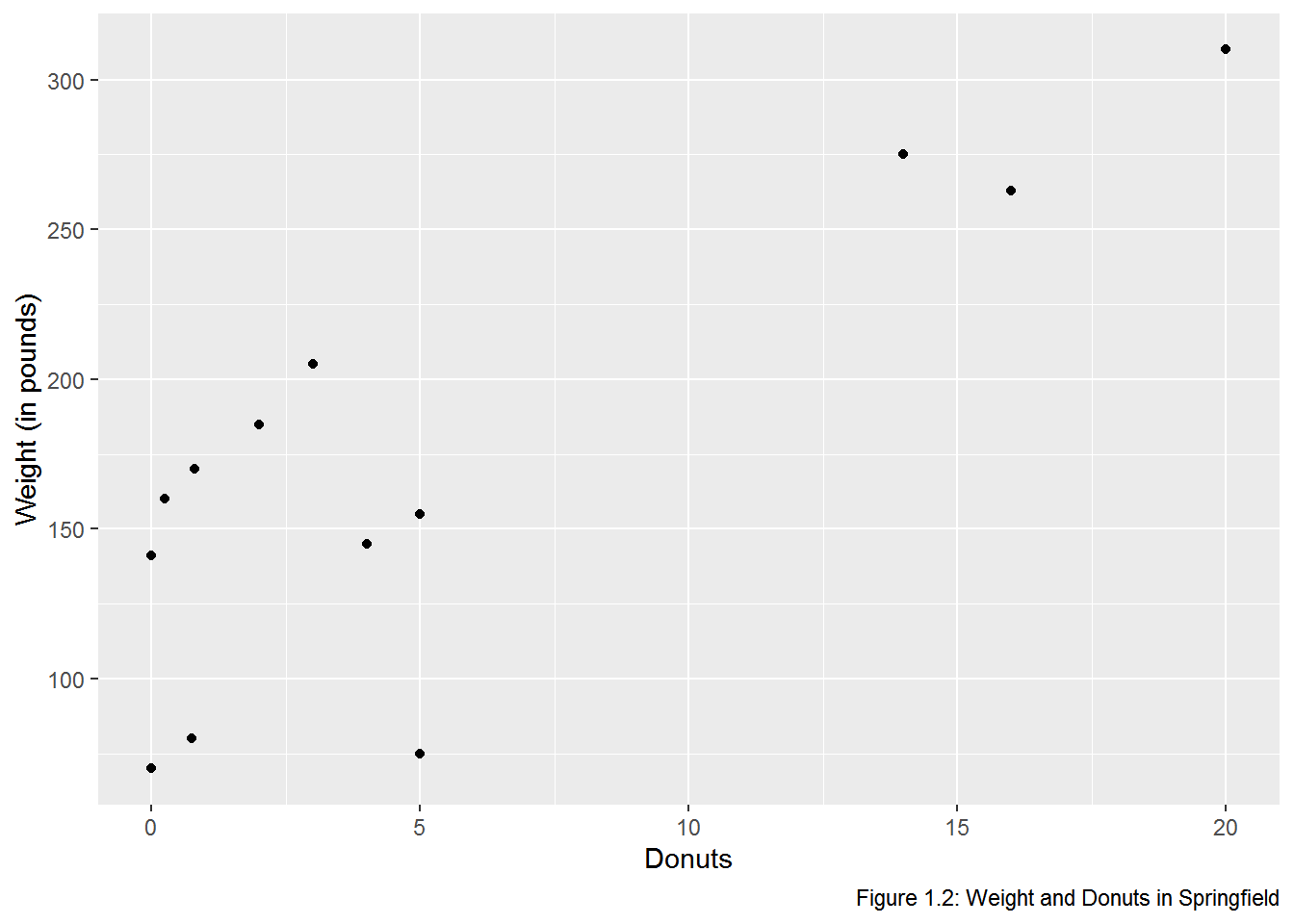# Add the verticle line at 0
(p3 <- p2 + geom_vline(xintercept = 0, color = "gray80", size = 1.25))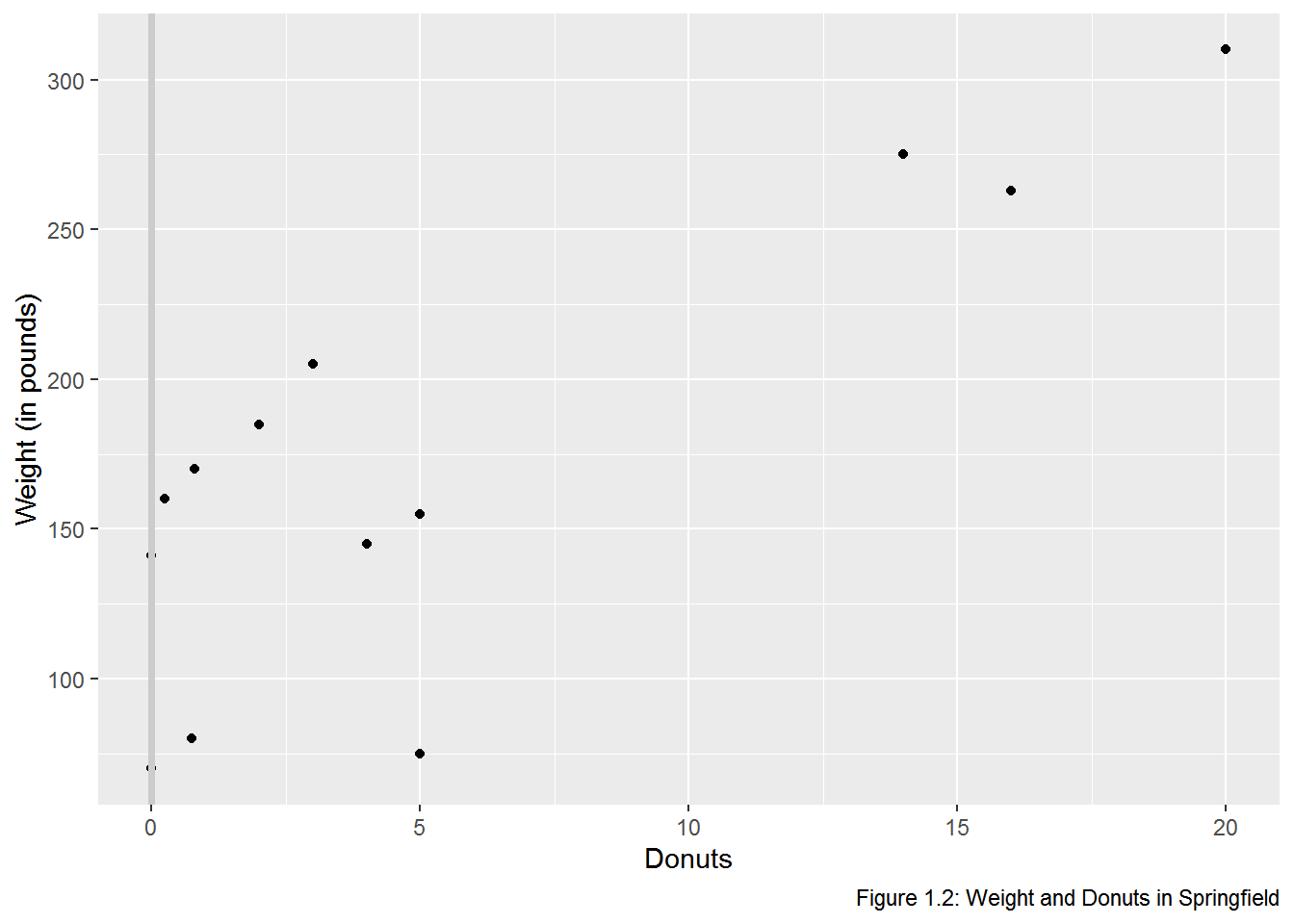# the layering effect puts the line in front of the points, so we have to add it before geom_point
(p4 <- p2 + #indentation makes to code easier to audit
geom_vline(xintercept = 0, color = "gray80", size = 1) +
geom_point())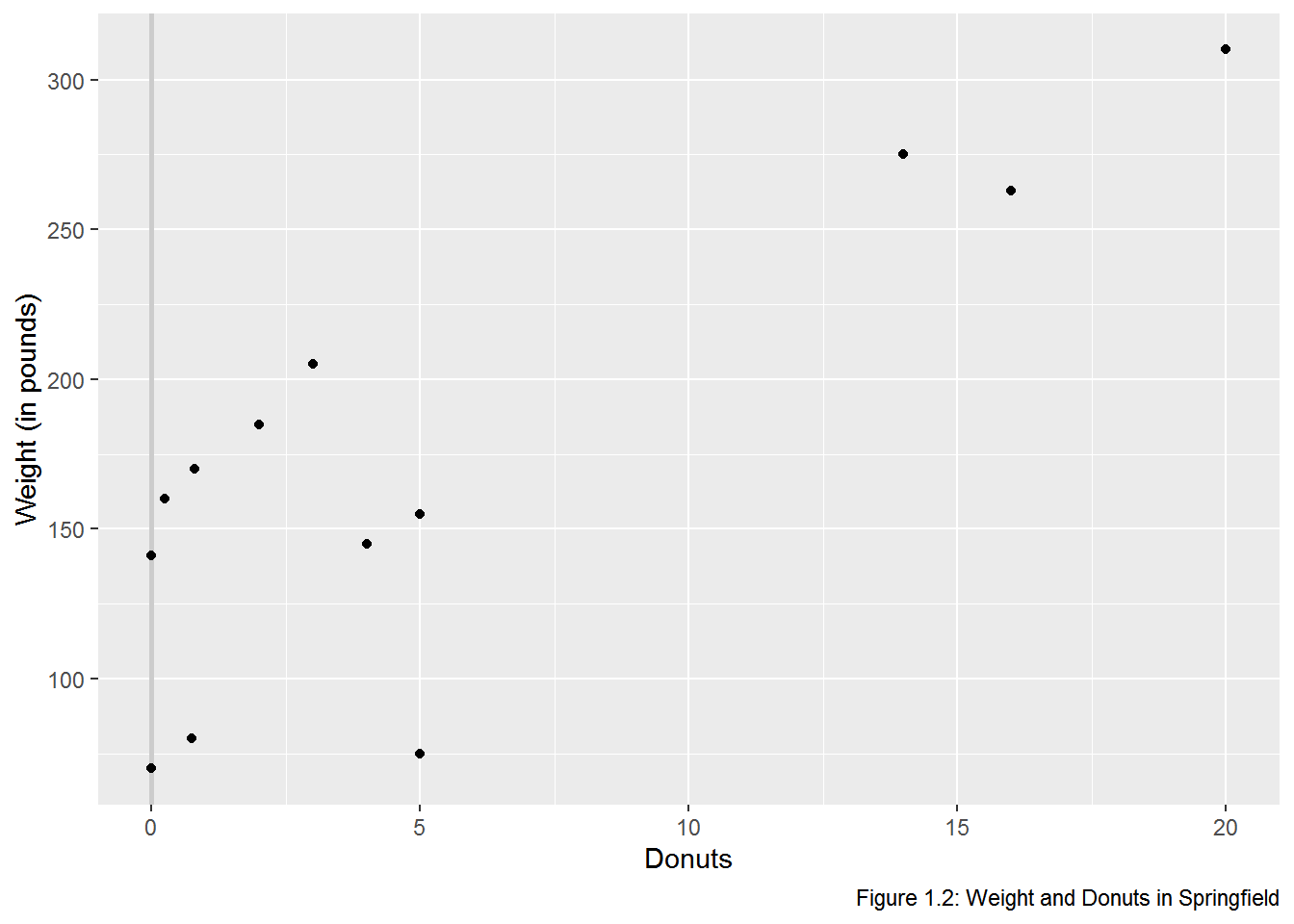# Add the name labels
(p5 <- p4 + geom_text(aes(label = name)))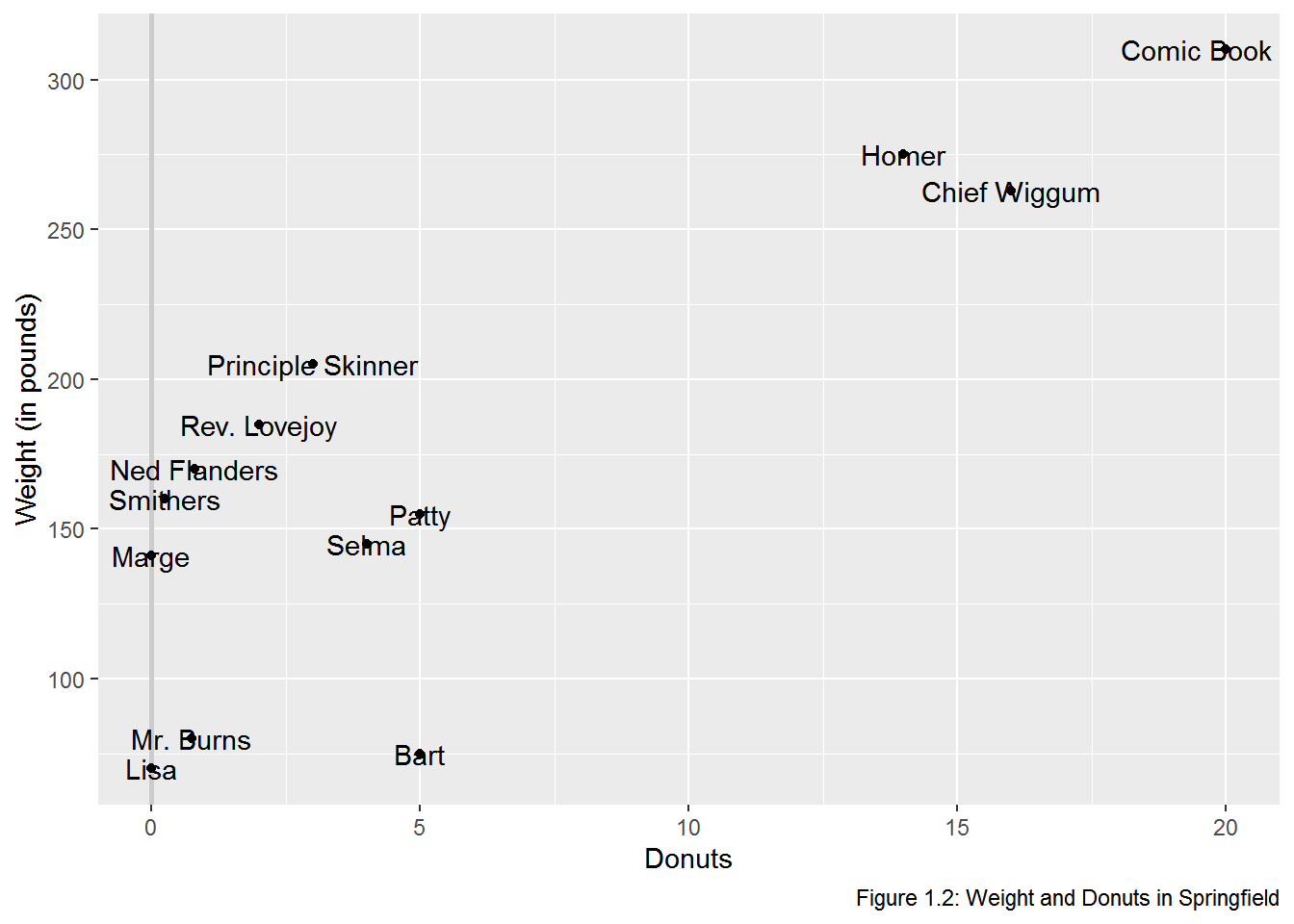# Clean up the name labels with the ggrepel package
library(ggrepel)
(p6 <- p4 + geom_text_repel(aes(label = name)))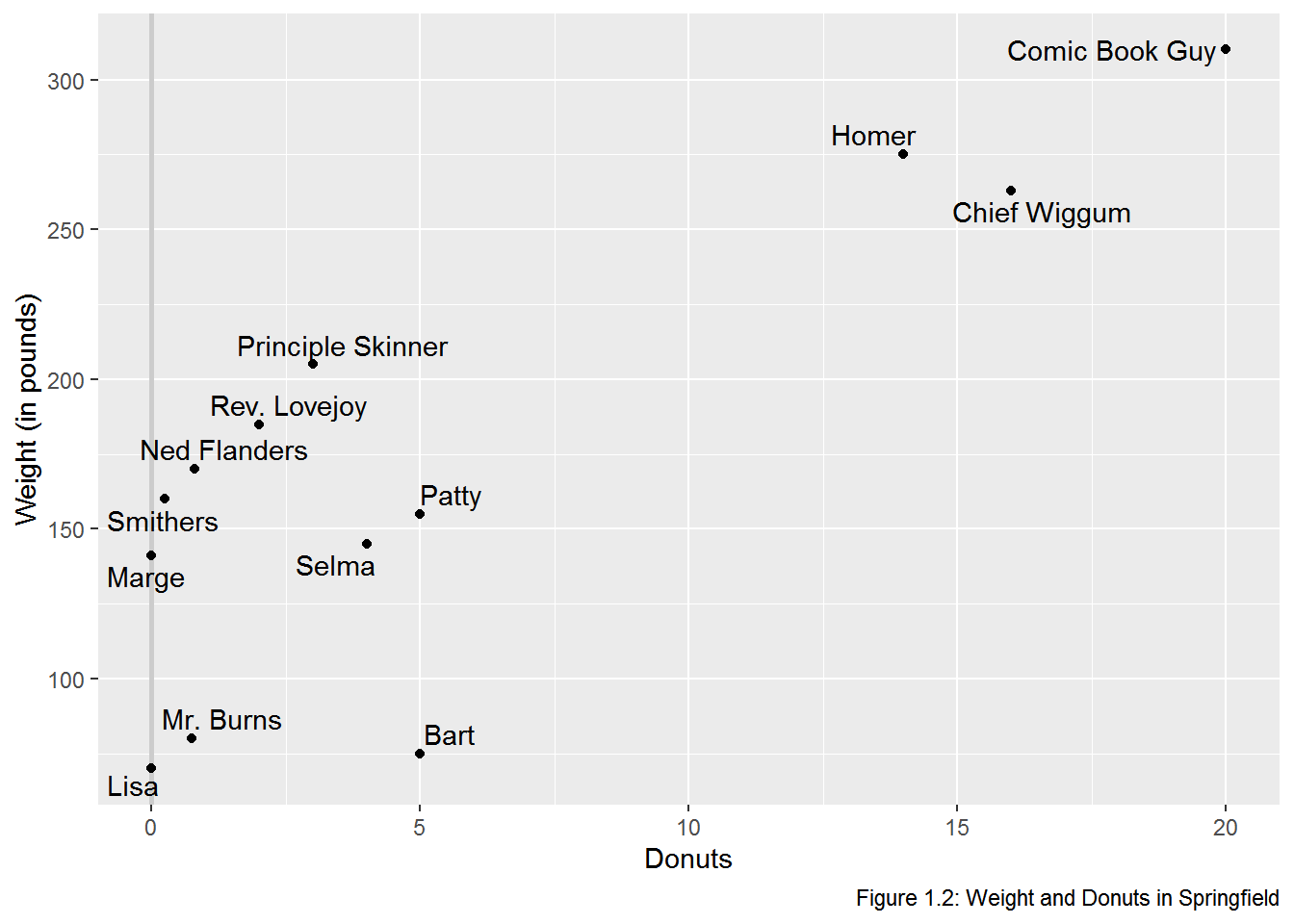# Use theme to adjust the non data elements
# \n in the y label creates a new line
(p7 <- p6 + labs(y = "Weight\n(in pounds)") +
theme(axis.title.y = element_text(angle = 0), # change orientation of y-axis label
panel.grid = element_blank(), # remove the background grid
panel.background = element_blank(), # remove the background
axis.line = element_line(), # add x and y axes
plot.caption = element_text(hjust = 0))) #move the caption to the left.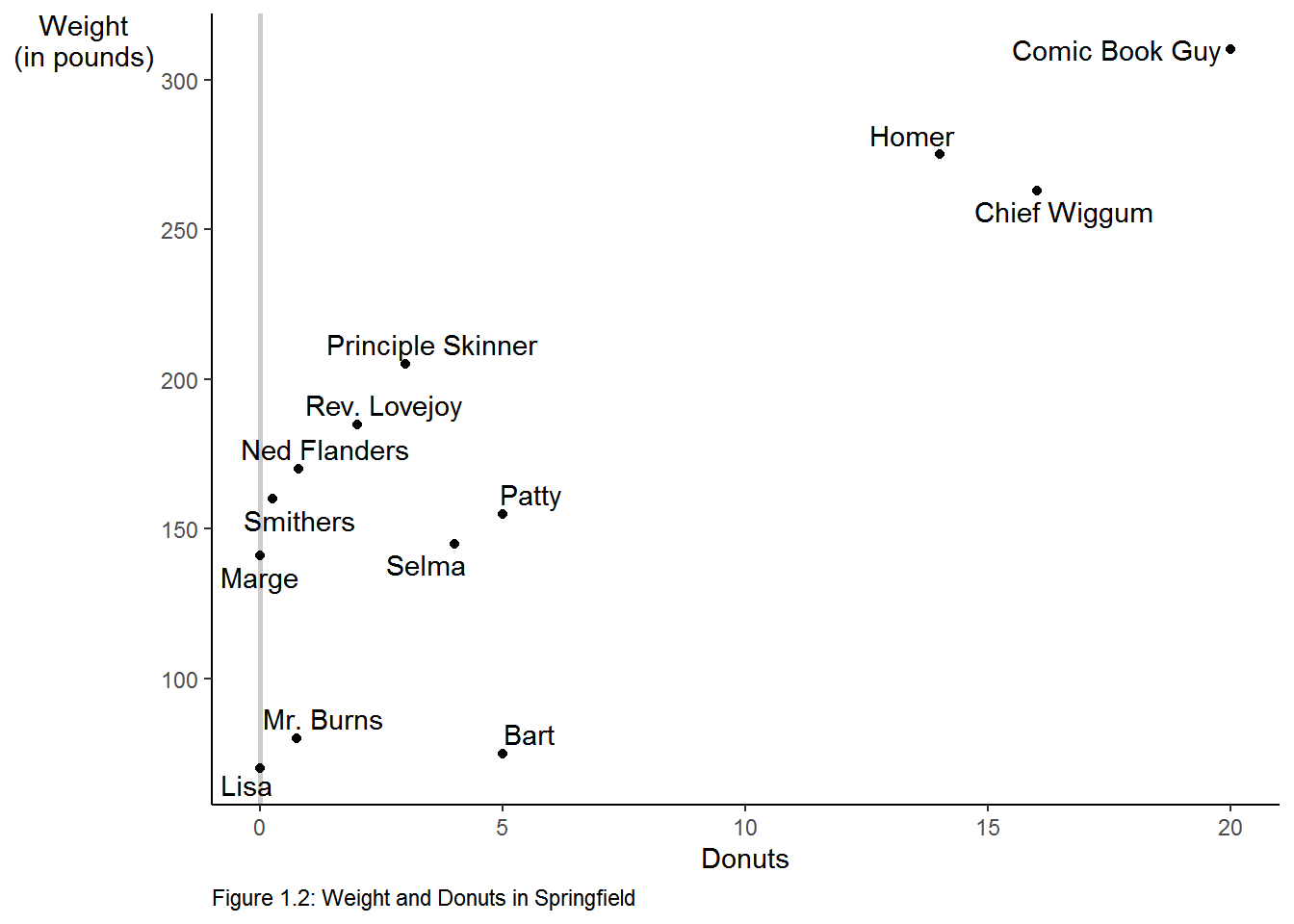We can make the graph in one step, if we desire.

p <- ggplot(data = donuts,
mapping = aes(x = donuts_per_week, y = weight)) +
geom_vline(xintercept = 0, color = "gray80", size = 1) +
geom_point() +
labs(x = "Donuts",
y = "Weight\n(in pounds)", # \n creates a new line
caption = "Figure 1.2: Weight and Donuts in Springfield") +
geom_text_repel(aes(label = name)) +
theme(axis.title.y = element_text(angle = 0),
panel.grid = element_blank(),
panel.background = element_blank(),
axis.line = element_line(),
plot.caption = element_text(hjust = 0))
p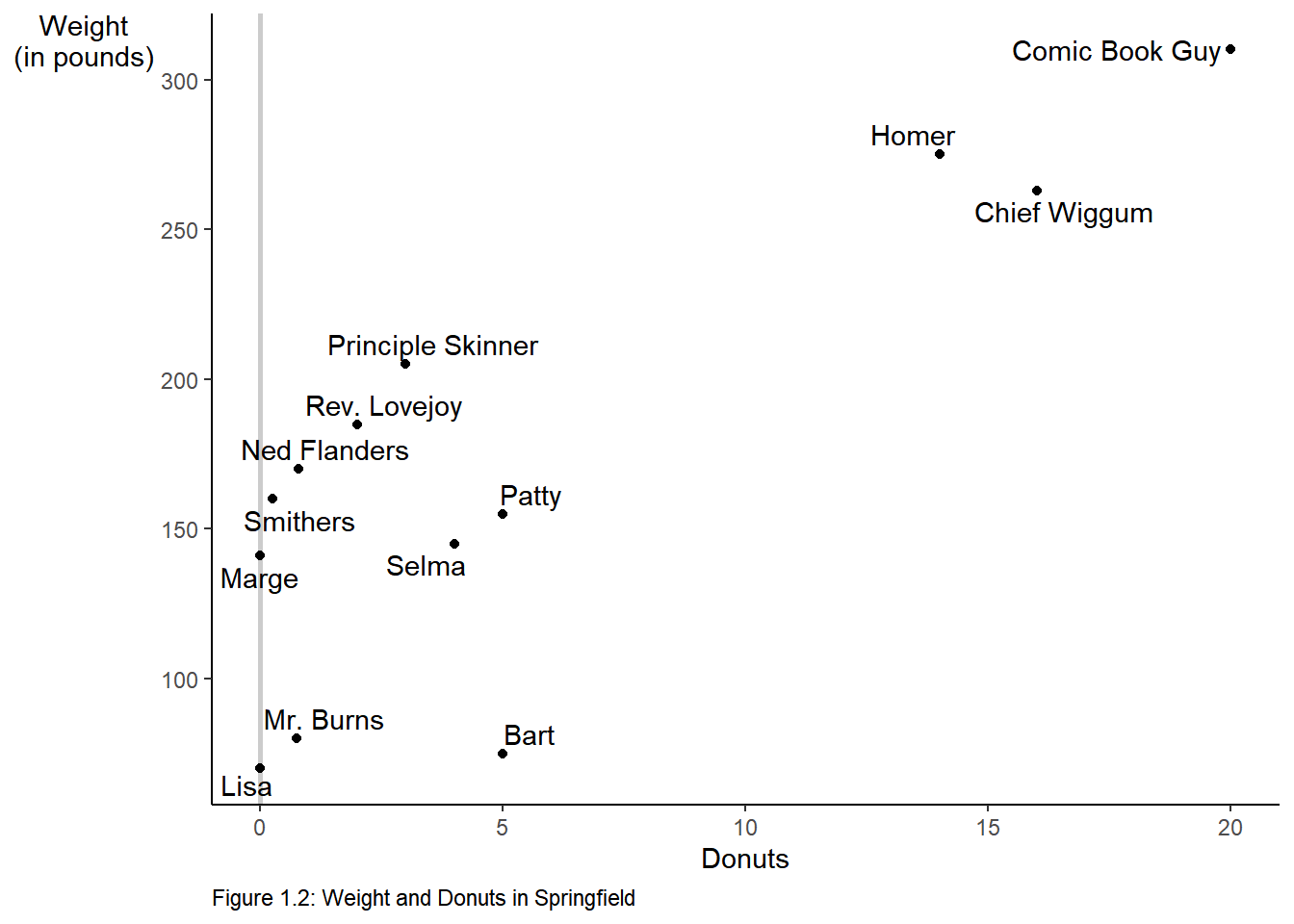### 3.1.3 Figure 1.3

p + labs(caption = "Figure 1.3: Regression Line for Weight and Donuts in Springfield") +
geom_smooth(method = "lm", se = F) + # method specifies the fit,  se = F turns off the error band.
annotate("text", label = expression(beta*" (the slope)"), # text annotation
y = 205, # position of the text
x = 8,   # position of the text
angle = 20, # angle of the text
color = "Blue") + # color of the text
geom_segment(aes(y = 121.613, x = 0, xend = 0, yend = 0),
color = "blue",
linetype = "dotted",
size = 1) +
annotate("text", label = expression(beta*" = 121.613"),
y = 115, x = 1.75, size = 3.5, color = "blue")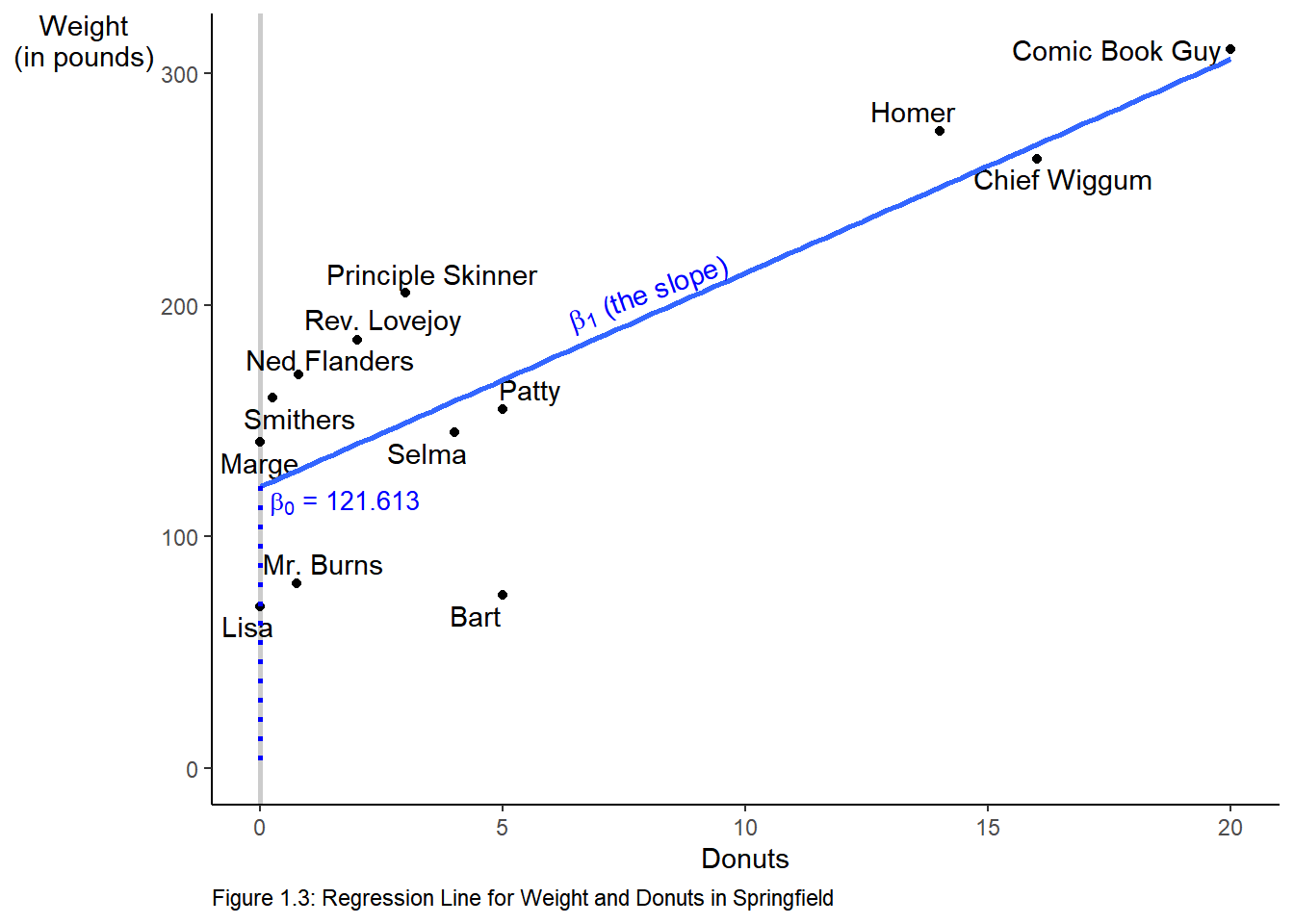1. You need install a package only once

2. You must load the package during each R session to make use of it.